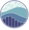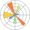# 2D Density Chart

This section explains how to build a 2d density chart or a 2d histogram with python. Those chart types allow to visualize the combined distribution of two quantitative variables. They can be build with `Matplotlib` or `Seaborn`.

## 💡 What is a 2D density chart?

There are several chart types allowing to visualize the distribution of a combination of 2 numeric variables. They always have a variable represented on the X axis, the other on the Y axis, like for a scatterplot (left).

Then the number of observations within a particular area of the 2D space is counted and represented with a color gradient. The shape can vary: hexagones result in a `hexbin chart`, squares in a `2d histogram`. A kernel density estimate can be used to get a `2d density plots` or a `contour plots`Cheat sheet: line customization with matplotlib

Confusing? Visit data-to-viz to clarify..

##Contour plot with `Seaborn`

The contour plot can be easily built thanks to the `kdeplot()` function of the Seaborn library.

##2D histogram with `Seaborn`

Build a 2d histogram thanks to the `hist2d()` function of the `Seaborn` library. Do not forget to play with the `bins` argument to find the value representing the best your data.

##Hexbin chart with `Matplotlib`

Split the graph area in hexagones and you get a hexbin density chart. This time, it is `matplotlib` that gets you covered thanks to its `hexbin()` function.

##2d density chart with `Matplotlib`

2D densities are computed thanks to the `gaussian_kde()` function and plotted thanks with the `pcolormesh()` function of `matplotlib()`.

##2d density and marginal plots

2D densities often combined with marginal distributions. It helps to highlight the distribution of both variables individually. It is pretty straightforward to add thanks to the `jointplot()` function of the `Seaborn` library.

## Contact

👋 This document is a work by Yan Holtz. You can contribute on github, send me a feedback on twitter or subscribe to the newsletter to know when new examples are published! 🔥